# 0、算法概述

## 0.1 算法分类

### 十种常见排序算法可以分为两大类：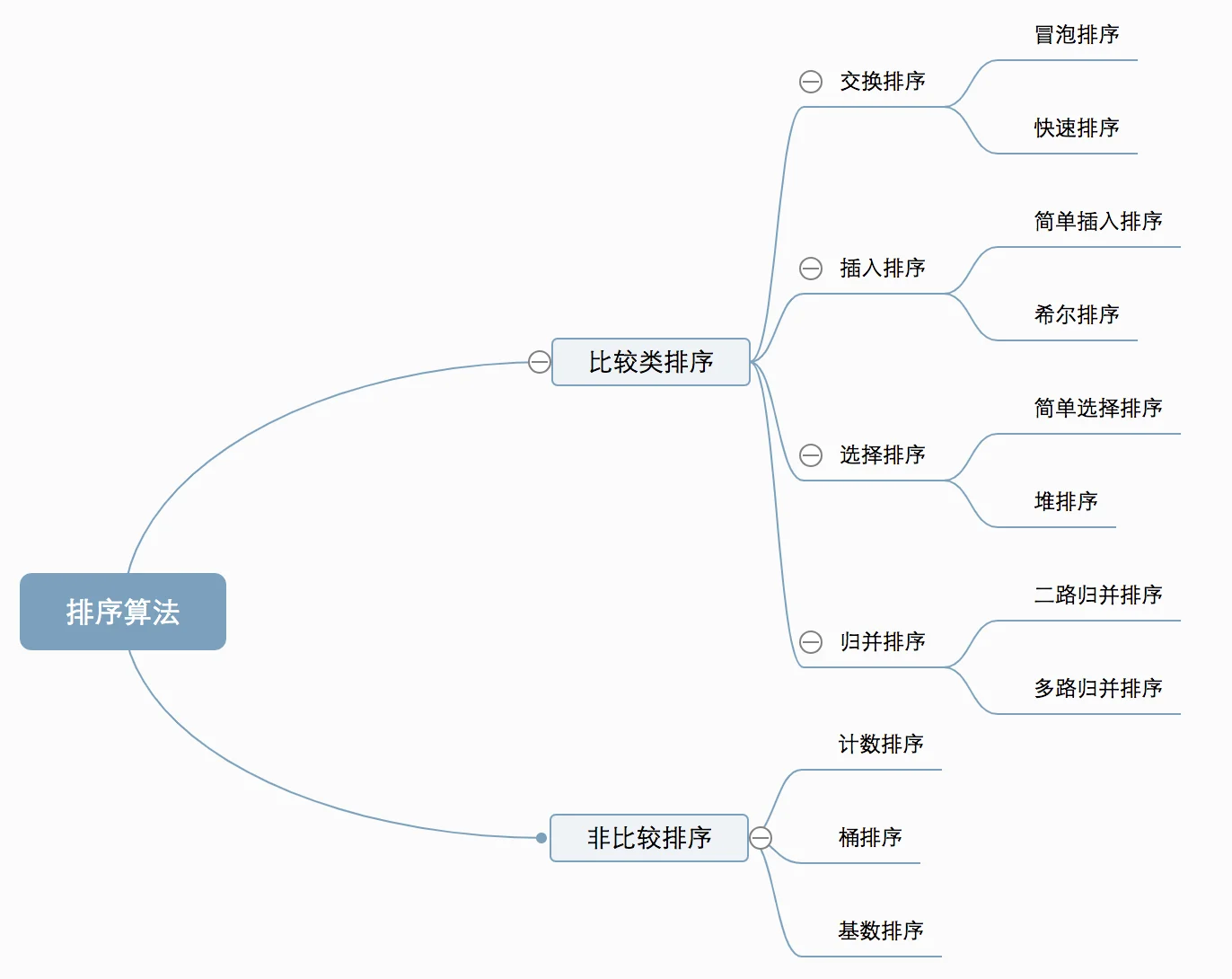### 0.2 算法复杂度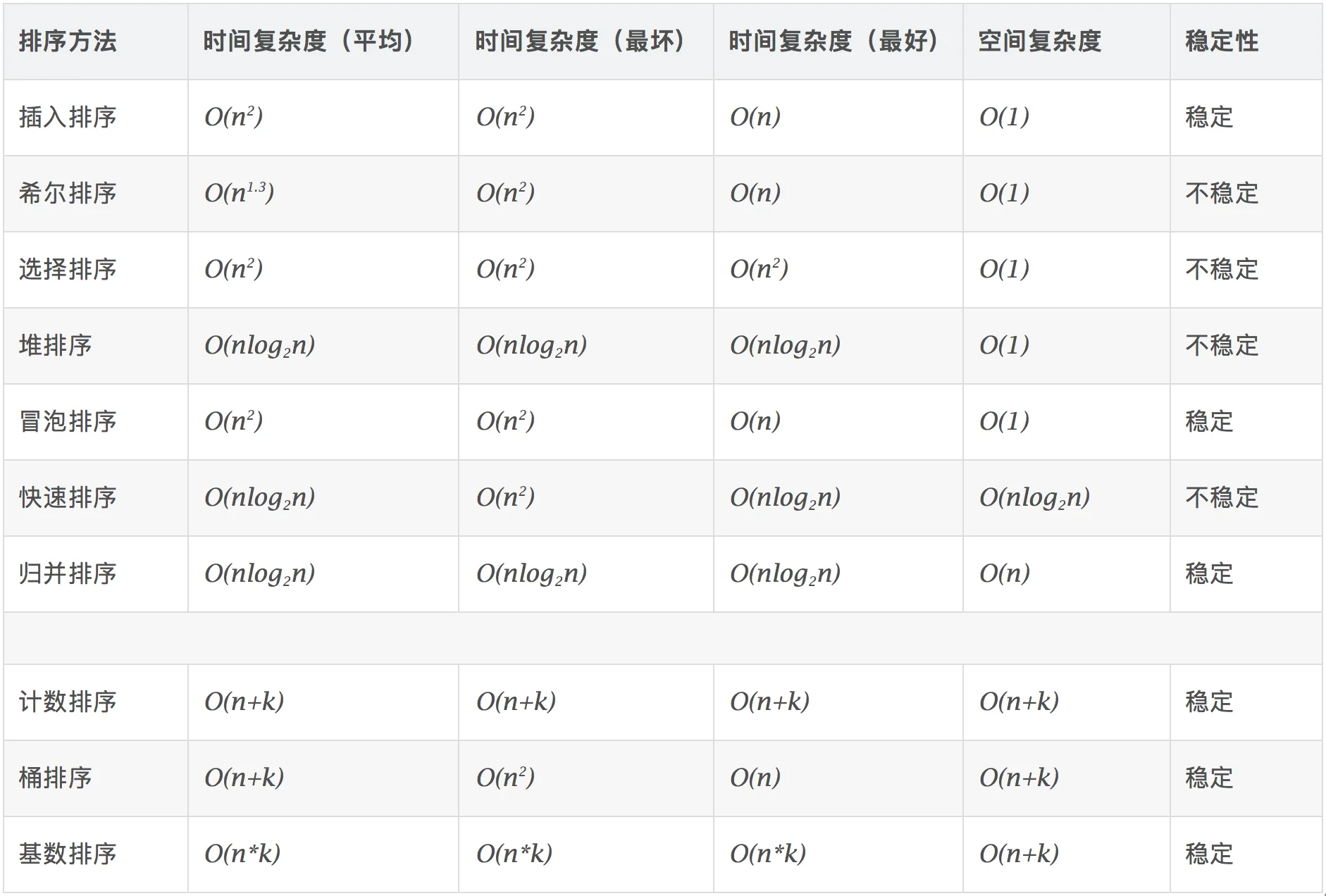## 1、冒泡排序（Bubble Sort）

### 1.2 动图演示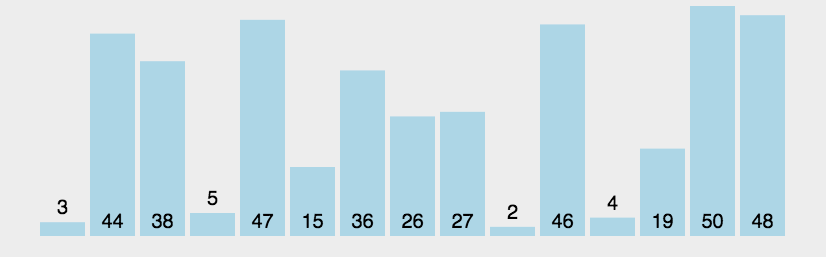### 1.3 代码实现

```package cn.fuqiang.arithmetic;

import java.util.Comparator;

/**
* 冒泡排序
* @Title: BubbleSort.java
* @Package cn.fuqiang.arithmetic
* @Description 冒泡排序 实现
* @date 2019年11月26日
*/
public class BubbleSort {
public static void main(String[] args) {
int[] arr = {4,1,5,3,8,10,28,2};
sort(arr);
so(arr);

Integer[] arr2 = {4,1,5,3,8,10,28,2};
sort(arr2,(a,b)-> b.compareTo(a));
so(arr2);
}
/**
* 冒泡排序 (特点：从后往前)
* 原理：前后元素两两之间进行比较 把大的往后扔
* 升序 (从后往前，从大到小)
* 外层循环是控制内层循环的次数，也就是说外层循环每循环一遍，让内层循环每次循环的次数减一次
* 这样就保证内层循环每次循环结束后最后面一个都是最大
* 例如 ： 3 6 4 1
* 外循环第一次 结束后，内循环 会把6放到最后面 3 4 1 6
* 外循环第二次开始时，内循环的循环次数会减一 也就是说 6 不会在参与比较
* 外循环第二次结束时， 内循环 会把4放到最后面 3 1 4
* 以此类推。。。。
* 外循环第三次结束时 内循环 1 3 4
* .。。。。 1 3
* .。。。。 1
* 最终结果 1 3 4 6
* @param t
*/
public static void sort(int[] t) {
for(int i=0;i<t.length;i++) {
int temp;
for(int j = 0;j<t.length-1-i;j++) { if(t[j]>t[j+1]) {
temp = t[j];
t[j]=t[j+1];
t[j+1]=temp;
}
}
}

}

/**
* 冒泡排序 对象类型排序
* 对象必须实现Comparator接口
* @param t 要遍历的数组
* @param comparator 定制的遍历规则
*/
public static void sort(T[] t,Comparator<? super T> comparator) {
for(int i = 0;i<t.length-1;i++) {
T temp;
for(int j = 1;j<t.length-i;j++) {
if(comparator.compare(t[j-1], t[j])<0) { temp = t[j-1]; t[j-1]=t[j]; t[j]=temp; } } } } /** * 传入任意数组或对象 输出到控制台 * @author 王福强 * @Description 前提是传入的对象必须重写 toString()方法 * @date 2018年6月8日 下午4:26:06 * @param obj */ public static void so(Object obj) { String str = ""; if(obj instanceof int[]) { int[] arr = (int[])obj; StringBuilder sb = new StringBuilder(); sb.append("["); for (int i : arr) { sb.append(i+","); } str = sb.substring(0,sb.lastIndexOf(","))+"]" ; }else if(obj instanceof long[]) { long[] arr = (long[])obj; StringBuilder sb = new StringBuilder(); sb.append("["); for (long i : arr) { sb.append(i+","); } str = sb.substring(0,sb.lastIndexOf(","))+"]" ; }else if(obj instanceof char[]) { char[] arr = (char[])obj; StringBuilder sb = new StringBuilder(); sb.append("["); for (char i : arr) { sb.append(i+","); } str = sb.substring(0,sb.lastIndexOf(","))+"]" ; }else if(obj instanceof short[]) { short[] arr = (short[])obj; StringBuilder sb = new StringBuilder(); sb.append("["); for (short i : arr) { sb.append(i+","); } str = sb.substring(0,sb.lastIndexOf(","))+"]" ; }else if(obj instanceof double[]) { double[] arr = (double[])obj; StringBuilder sb = new StringBuilder(); sb.append("["); for (double i : arr) { sb.append(i+","); } str = sb.substring(0,sb.lastIndexOf(","))+"]" ; }else if(obj instanceof float[]) { float[] arr = (float[])obj; StringBuilder sb = new StringBuilder(); sb.append("["); for (float i : arr) { sb.append(i+","); } str = sb.substring(0,sb.lastIndexOf(","))+"]" ; }else if(obj instanceof Object[]) { Object[] arr = (Object[])obj; StringBuilder sb = new StringBuilder(); sb.append("["); for (Object i : arr) { sb.append(i.toString()+","); } str = sb.substring(0,sb.lastIndexOf(","))+"]" ; }else { str=obj.toString(); } System.out.println(str);; } }```

## 2、选择排序（Selection Sort）

### 2.1 算法描述

n个记录的直接选择排序可经过n-1趟直接选择排序得到有序结果。具体算法描述如下：

• 初始状态：无序区为R[1..n]，有序区为空；
• 第i趟排序(i=1,2,3…n-1)开始时，当前有序区和无序区分别为R[1..i-1]和R(i..n）。该趟排序从当前无序区中-选出关键字最小的记录 R[k]，将它与无序区的第1个记录R交换，使R[1..i]和R[i+1..n)分别变为记录个数增加1个的新有序区和记录个数减少1个的新无序区；
• n-1趟结束，数组有序化了。

### 2.2 动图演示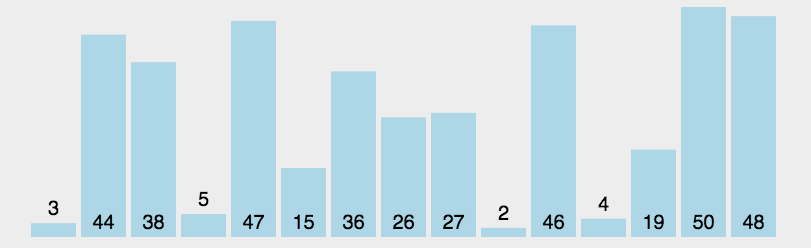### 2.3 代码实现

```package cn.fuqiang.arithmetic;

import java.util.Comparator;

/**
* 选择排序  的两种实现方式
* (在这里要注意，因为最后一个位置的数绝对是最后循环后最大的值，
* 所以外层循环的循环次数 最好是arr.length-1).
* @Title: SelectionSort.java
* @Package cn.fuqiang.arithmetic
* @Description
* @date 2019年11月26日
*/
public class SelectionSort {
public static void main(String[] args) {
int[] arr = {4,1,5,3,8,10,28,2};
sort(arr);
so(arr);

Integer[] arr2 = {4,1,5,3,8,10,28,2};
sort(arr2,(a,b)-> b.compareTo(a));
so(arr2);
}

/**
* 选择排序   第一种实现方式  (赋值)    升序   （特点 ：从小到大）
* 原理: 每次把最小的都放在前面，
* 外层循环控制每次遍历的次数，内层循环替换每次找出来的最小值，放在  arr[i]的位置
* 这样当内层比较和替换的时候，就保证每次最小的都在最前面
* 例如：3 2 6 1
* 第一次外层循环为  i 为  0    第一个就是最小的值放最小的值
* 内层循换会把每次找出的最小值放在arr 的位置
* 第二次外层循环为 i 为 1
* 内层循环会把每次找出最小的值放在 arr 的位置
*
* 外循环    第一次   i=0
* 内部循环
* 第一次  2 3 6 1
* 第二次  2 3 6 1
* 第三次  1 3  6 2
*
* 外循环    第二次   i=1
* 内部循环
* 第一次  3 6 1
* 第二次  1 6 3
*
* 外循环    第三次   i=2
* 内部循环
* 第一次  3  6
*
*
* @Description
* @date 2019年11月26日
* @param arr int类型数组
*/
public static void sort(int[] arr) {
for(int i = 0;i<arr.length-1;i++) {
int temp;
for(int j = i+1 ; j arr[j]) {
temp = arr[i];
arr[i] = arr[j];
arr[j] = temp;
}
}
}
}

/**
* 选择排序  第二种实现(记录下标)  升序   （特点 ：从小到大）
* 每次循环记录最小的下标 min 最后跟 arr[i] 位置的下标交换位置
* 这样当外层循环每次循环一次 会先记下  arr[i] 的下标，
* 然后让内层循环从 i+1 开始比较去比较  arr[min] 与 arr[j]
* 如果 min下表对应的值 比  j下标对应的值大， 则记录 j的下标    min  = j
* 然后拿新的 min下标去跟后面比较，依此类推。
* 当内层循环循环结束后记录的下标是剩余数中最小的呢个值的下标
* 然后用一个零时变量将他们的值交换过来，就算本身是最小的，也是替换自己
* 例如  1 3 6 2
*
* 第一次外层循环结束后
*  1 3 6 2
* 第二次外循开始时，会记录i的下标
* 然后开始内层循环，会记录2的下标
* 内层循环结束后，会把  3 与 2的位置调换
* 2 6 3
* 。。。。。
* 。。。。
* 3 6
*
* @Description
* @date 2019年11月26日
* @param arr
* @param comparator
*/
public static void sort(T[] arr,Comparator comparator) {
for(int i = 0;i<arr.length-1;i++) {
int min=i;
T temp=null;
for(int j = i+1 ; j

```

## 3、插入排序（Insertion Sort）

### 3.1 算法描述

• 从第一个元素开始，该元素可以认为已经被排序；
• 取出下一个元素，在已经排序的元素序列中从后向前扫描；
• 如果该元素（已排序）大于新元素，将该元素移到下一位置；
• 重复步骤3，直到找到已排序的元素小于或者等于新元素的位置；
• 将新元素插入到该位置后；
• 重复步骤2~5。

### 3.2 动图演示

```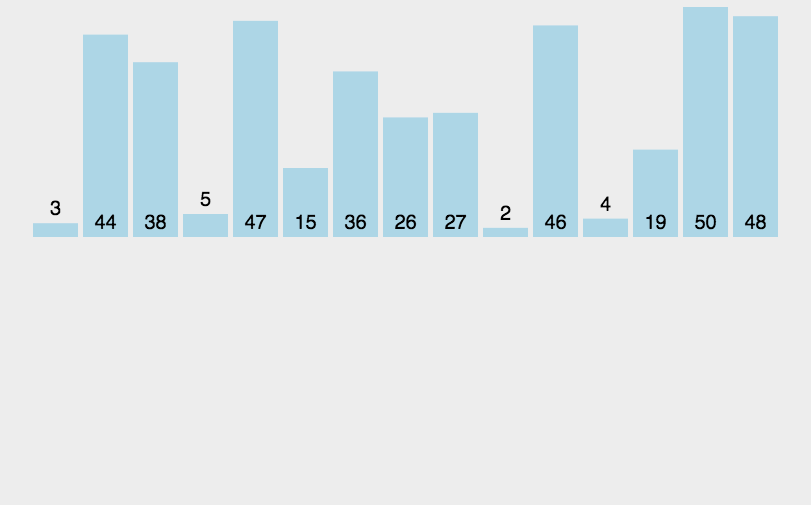```

#### 3.2 代码实现

```package cn.fuqiang.arithmetic;

import java.util.Comparator;

/**
* 插入排序
* @Title: InsertionSort.java
* @Package cn.fuqiang.arithmetic
* @Description
* @date 2019年11月26日
*/
public class InsertionSort {
public static void main(String[] args) {
int[] arr = {4,1,5,3,8,10,28,2};
sort(arr);
so(arr);

Integer[] arr2 = {4,1,5,3,8,10,28,2};
sort(arr2,(a,b)-> b.compareTo(a));
so(arr2);
}
/**
* 插入排序   升序(特点:拿当前下标值与前面的值比较，将小的值排在对应的位置，把大的往后移)
* 循环从 i=1  开始      用temp记录当前位置的值arr[i]，
* 然后设置内部循环控制下标，通过循环将下标每次减一。
* 然后判断temp与arr[index]的大小， 如果temp小于arr[index]则将arr[index-1]值移动到下一个位置，
* 然后将下标往前挪一位 也就是 index-1 (因为第一次比较时记录了 temp = arr[i] 所以不用担心会将值覆盖)
* 如果不进入内循环则说明，下标已经移动到最前面了没有比该值更小的了，
* 这时候就把记录的 temp 赋值给  index+1 的位置上(因为index已经减到前一个了)
* 比较第一个位置 和第二个位置的
* 例如  3 1 6 2
* 外循环第一次  i=1
* 外层循环直接从  下标为一的开始
* 比较1 与3的大小，然后把3的位置移动到后面一位，
* 应为内层循环index--后小于0了，所以不在循环，
* 然后因为把3移动到后面一位的时候前面空了一个位置，所以就把1插入
* 1 3 6  2
* 。。。。
* 外循环第三次  i=3
* 比较  2 与 6 然后把6往后移
* 比较  2与3把三往后移
* 然后比较2与1    把 2 插入到空缺位置
*
* @Description
* @date 2019年11月26日
* @param arr
*/
public static void sort(int[] arr) {
for(int i = 1 ; i < arr.length ; i ++) { int index = i-1; int temp = arr[i]; while(index>=0 && arr[index]>temp) {
arr[index+1] = arr[index--];
}
arr[index+1] = temp;
}
}

/**
* 对任意对象排序
* @Description
* @date 2019年11月26日
* @param arr
* @param comparator
*/
public static void sort(T[] arr,Comparator comparator) {
for(int i = 1;i<arr.length;i++) { T temp=arr[i]; int index; for(index = i-1;index>=0 && comparator.compare(arr[index], temp)<0;index--) {
arr[index+1]=arr[index];
}
arr[index+1] =temp;
}
}

/**
* 传入任意数组或对象   输出到控制台
* @Description  前提是传入的对象必须重写 toString()方法
* @date 2019年11月26日
* @param obj
*/
public static void so(Object obj) {
String str = obj.toString();
if(obj instanceof int[]) {
int[] arr = (int[])obj;
StringBuilder sb = new StringBuilder();
sb.append("[");
for (int i : arr) {
sb.append(i+",");
}
str = sb.substring(0,sb.lastIndexOf(","))+"]" ;

}else if(obj instanceof long[]) {
long[] arr = (long[])obj;
StringBuilder sb = new StringBuilder();
sb.append("[");
for (long i : arr) {
sb.append(i+",");
}
str = sb.substring(0,sb.lastIndexOf(","))+"]" ;
}else if(obj instanceof char[]) {
char[] arr = (char[])obj;
StringBuilder sb = new StringBuilder();
sb.append("[");
for (char i : arr) {
sb.append(i+",");
}
str = sb.substring(0,sb.lastIndexOf(","))+"]" ;
}else if(obj instanceof short[]) {
short[] arr = (short[])obj;
StringBuilder sb = new StringBuilder();
sb.append("[");
for (short i : arr) {
sb.append(i+",");
}
str = sb.substring(0,sb.lastIndexOf(","))+"]" ;
}else if(obj instanceof double[]) {
double[] arr = (double[])obj;
StringBuilder sb = new StringBuilder();
sb.append("[");
for (double i : arr) {
sb.append(i+",");
}
str = sb.substring(0,sb.lastIndexOf(","))+"]" ;
}else if(obj instanceof float[]) {
float[] arr = (float[])obj;
StringBuilder sb = new StringBuilder();
sb.append("[");
for (float i : arr) {
sb.append(i+",");
}
str = sb.substring(0,sb.lastIndexOf(","))+"]" ;
}else if(obj instanceof Object[]) {
Object[] arr = (Object[])obj;
StringBuilder sb = new StringBuilder();
sb.append("[");
for (Object i : arr) {
sb.append(i.toString()+",");
}
str = sb.substring(0,sb.lastIndexOf(","))+"]" ;
}else {
str=obj.toString();
}
System.out.println(str);;
}

}

```

### 3.4 算法分析

1. #1这么强

建站：游海原1年前 (2019-11-28)回复### 觉得文章有用就打赏一下文章作者

#### 支付宝扫一扫打赏#### 微信扫一扫打赏Vieu4.5主题• #### anhuahua20Q Q 登 录